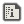# Making a smooth Jump for platformersAutz64Created:
Here i'm going to show how to perform a smooth jump for 2D platforms. The tutorial will kinda fall short, because this isn't too complicated. At this point you should be familiarized with how to move sprites on screen using screen coordinates. For this tutorial i'm going to use the following code:
```SPSET 0,0

PX=200
PY=120

WHILE 1
VSYNC 'VSYNC on top, because on bottom you will lose 1 frame

STICK OUT SX,SY
PX=PX+SX*4

SPOFS 0,PX,PY
WEND
```
This code will move the player on the X axis by using the stick. Now, let's start:

# 1.- Concepts

Gravity is a constant force that, on summary, push us down at a given speed (9.8 m/s). This should be enough, but to expand it a bit: A digital equivalent of that force would be a constant that acts like a force on a simple math operation. And when we jump, in real life i mean, what are we doing? We're applying a force that is bigger than the gravity in the same direction (down). And given Newton's third law, we ended up with a force on the opposite direction (up), son summary, our jump force negates gravity and allows us to go up instead. However, there's a detail: Gravity is a constant, but our jump force isn't. Which means that the jump force will slowly fade until we end up on the floor like we started. With all this info, we should be ready to wrap the logic behind all this and translate it into proper code.

# 2.- Everything else

## 2.1.- Gravity

First we need to setup gravity. And as explained ealier, is a constant, so the setup should be easy as following:
```G=5 'Gravity. It will move the player 5 pixels down on each frame
```
Then we need to apply that force to the player, which means we need to toy around with the player's Y axis like this:
```PY=PY+G

'Small tweak to avoid falling into oblivion
IF PY>220 THEN PY=220

SPOFS 0,PX,PY
```
When executing the code, the player will fall down until PY is bigger than 220, that it will serve as a imaginary floor for this tutorial. Now, the gravity is all done.

## 2.2.- Jumping

Now, as explained ealier, we need to setup a temporal force that will act as a gravity negator. It should be like this:
```J=0
```
Now, we need to do three(3) things here: 1) initialize J when the player press a button (jump button) using a value that is bigger than G 2) include J into the PY math 3) decrease J over time For all that, i'm going to be simple:
```'1) Complete
IF BUTTON(2) AND #A THEN J=15

'2) Complete
PY=PY+(G-J) 'If J is bigger than G, which is the natural way to do it, the result will be negative. And thus, pushing the player up.
SPOFS 0,PX,PY

'3) Complete
IF J>0 THEN J=J-1
```
Now, the final code should look like this:
```SPSET 0,0

J=0
G=5

PX=200
PY=120

WHILE 1
VSYNC

IF BUTTON(2) AND #A THEN J=15

STICK OUT SX,SY
PX=PX+SX*4
PY=PY=(G-J)

IF PY>220 THEN PY=220

SPOFS 0,PX,PY

IF J>0 THEN J=J-1
WEND
```
And this should be all! Of course, by the nature of doing this, a lot of tweaks needs to be made. Like avoiding jumping more than once, and using proper floor and platformers. But that's up to you guys!

No posts yet (will you be the first?)